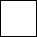Skill 6D Missing Addends Here, you can see how learning the previous skill of fact families can help with the skill of missing addends. For example, you can use a related subtraction fact to find the missing addend in the problem below. So, to find the answer to 6 + ___ = 14, you can use the problem 14 - 6 =____. By the way, while the term "sum" means the answer to an addition problem, the term "addend" describes the actual numbers that are being added in the problem itself. What related subtraction fact should you use to find the answer to the missing addend problem?   Write the related subtraction fact on a separate paper. 6 += 14 - =